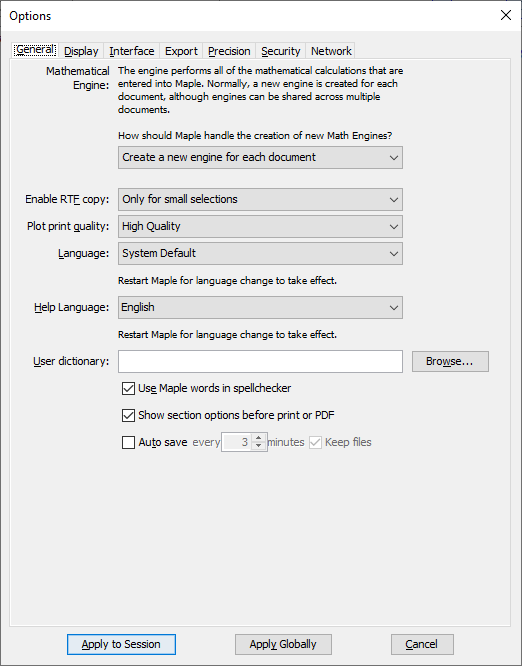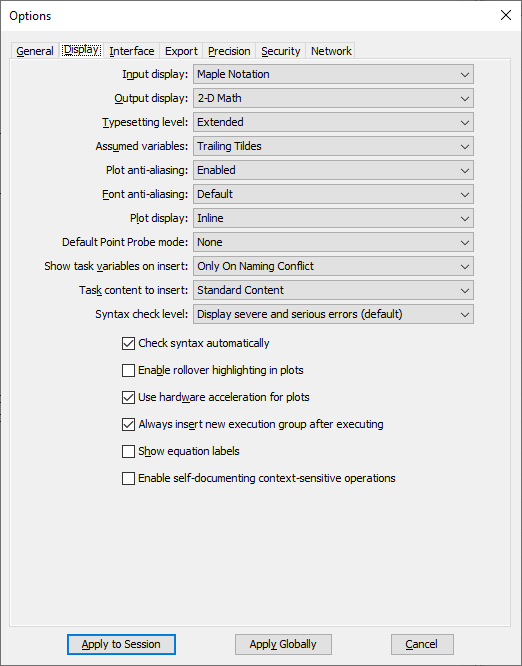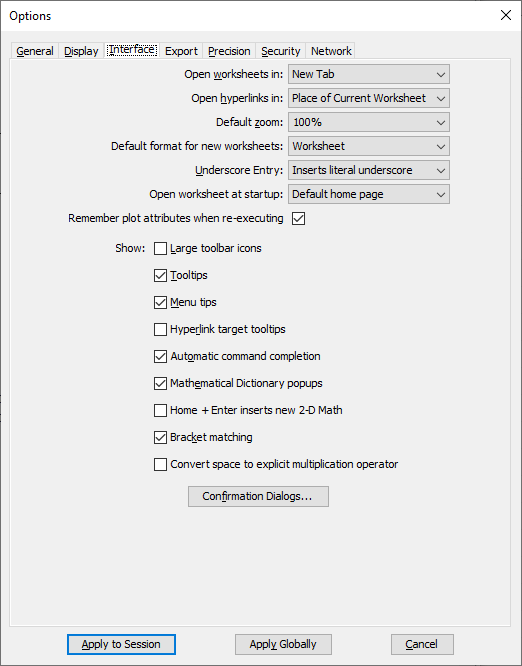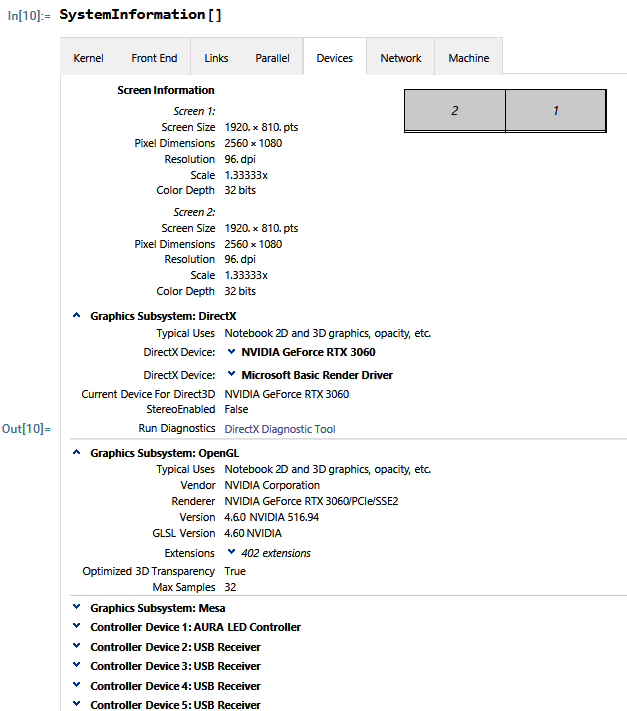## Maple 2023 networktool installation problem...

I know that this is not really a question regarding core Maple package, but I am running into problems during the installation process.

After having upgraded Maple networktools as mentioned, I am unable to run the activation program due to an error.

"Java Virtual Machine Launcher: Error: Could not create the Java Virtual Machine."

I've never had that problem before in previous versions. There was no Java installed on the (virtual) server, so I installed the latest OpenJDK to check if that solved the problem.

Unfortunately it didn't.

Any hints would be appreciated.

Windows Server 2012R2

## strange font problem with Maple 2023 on windows 10...

My main question is: How to change the font used in worksheet by Maple for 1D input from Courier to another font say times new roman? Is there a setting for this so it applies all the time?

ps. I found Can-I-Change-the-Default-Fonts-or-Style-for-Maple-Worksheets-and-Documents?language=en_US  (very hard to follow and confusing, but it seems that is only way to fix this problem now is to change the default font).

-----------------------------------------------------------------------------------------------------------------

I noticed strange font problem using Maple 2023 on windows 10. This problem does not show on Maple 2022.2 (at least I do not think I've seen it or noticed it before).

Variables with _ between the names, will have the underscore not display sometimes as I move the cursor around (movie at end).

When scrolling back up, the underscores no longer become visible.

But as I move the cursor over the variable name which containes the underscore, they will now show up.

I am sure this is a font issue. The zoom is set at 100%. I made no changes at all other than making the input 1D math as I normally do and set the default to worksheet. Some of my setting are below.

This could be a DPI issue settings of some sort. My monitor is standard monitor (not a 4K one) and again, I have not changed any settings on my PC after I installed Maple 2023 and did not change any hardware.Here is some system information alsoHere is the movie showing the problemAs I play more with it, I notice a common theme. This happens when I hit the UP ARROW to go to the line above. Then suddenly the underscroes no longer visible. Once I hit the LEFT arrow (now I am at the above line), they show up again.

Here is another movie which more clearly shows this.

This makes it very hard to work with the code in the worksheet. But I am sure this can be fixed as it looks like just a font configuration issue with Maple on windows. But I do not know what it is and how to fix it.I found something new. When setting the ZOOM at 125% instead of 100% as above, I notice the underscores do not completely disappear but become THINNER but one can still see them. This affects only the underscores. When the ZOOM at 150%, they do not change at all.  Here is a movie. first part at 125%. Notice how they become little thinner when doing the same thing as above. But at 150% they remain visible because they do not change thinkness. When going back to 100% zoom, they  no longer show.

So the problem is that they are there all the time, but depending on the ZOOM level, they beome thinner and thinner until they become invisible to the eye.I found some related issue on windows 10 with some application where this problem shows up.

some-characters-missing-or-cut-off-when-displayed-on-screen

"When you view your document in Microsoft Word, some characters may be missing, or the top (or bottom) of some characters may be cut off.

This problem may affect the following types of characters:

Underscore"

The above is about WORD, but it could as well apply to Maple?

I also found  underscore not rendering with courier

"Underscore characters are not rendered in new Google Docs in the Courier
New 11pt or 12pt fonts."

My question is: How to change the font used in worksheet by Maple? Now it is using Courier. May be if I change the font, this problem will go away?

Update: Found a workaround!  It is the font used!  I changed from Courier to Times New Roman and now the underscore do not change thinkness!  I just need a way to make this permenant for all 1D Maple input.   Here is a new movie showing it is fixed. You can see the underscores remain visible, all at 100% ZOOM level. Once I change to Courier, the problem show up again.

I am not sure if this is a Maple issue or windows.## Why is `ArrayTools[GeneralOuterProduct]` extremely...

The new command ArrayTools[GeneralOuterProduct] (introduced in Maple 2021) computes the generalized outer product of two rtables, and again, there exists a similar function Outer in Mma (cf. the end of this question). But in practice, it appears that this Maple command is not so fast as Mma's one. To begin with, we need to generate four lists: w, x, y, and z. Our goal is forming all possible combinations of the lowest‐level elements in a nested structure (rather than a flat structure). Now let us start the test.

In Mathematica (the real time is about ):And in Maple (the real time is about ):

 > restart;
 > w := [`\$`](0 .. 1e4): x := [`\$`](0 .. 2e3): y := [`\$`](0 .. 3e2): z := [`\$`](0 .. 4e1):
 >(1)
 >(2)
 >=As you can see, Maple and Mathematica returns identical results (∵p1p3); nevertheless, Maple consumes too much time: the ratio is 199.880/0.784176 ≈ 254.892. (What a wide gap between them!)
So, is there any possibility of speeding up Maple's `ArrayTools:-GeneralOuterProduct`? Or any ideas of obtaining the same results in Maple efficiently?

Explanatory notes. Here is an illustrative animation:## How do I apply a procedure at SPECIFIED levels?...

That is to say, a generalized `map`
E.g., here is a nested list:

`nl := [[[[s, t]], [u, [v, w]]], [[x, [y, z]]]]:`

We can use `map` to apply the mapped function F to "each operand" (i.e., the first‐level parts) of :

```:-map(F, nl);
=
[F([[[s, t]], [u, [v, w]]]), F([[x, [y, z]]])]

```

But in Mathematica, we can make further explorations:

```In:= nl = {{{{s, t}}, {u, {v, w}}}, {{x, {y, z}}}};

In:= Map[F, nl, {1}] (*Maple's result*)

Out= {F[{{{s, t}}, {u, {v, w}}}], F[{{x, {y, z}}}]}

In:= Map[F, nl, {2, -2}]

Out= {{F[{F[{s, t}]}], F[{u, F[{v, w}]}]}, {F[{x, F[{y, z}]}]}}

In:= Map[F, nl, {-3, 3}]

Out= {{F[{F[{s, t}]}], F[{F[u], F[{v, w}]}]}, {F[{F[x], F[{y, z}]}]}}

In:= Map[F, nl, {0, \[Infinity]}, Heads -> \[Not] True]

Out= F[{F[{F[{F[{F[s], F[t]}]}], F[{F[u], F[{F[v], F[w]}]}]}], F[{F[{F[x], F[{F[y], F[z]}]}]}]}]```

Note that the last case has been implemented in Maple as `MmaTranslator[Mma][MapAll]`:

```MmaTranslator:-Mma:-MapAll(F,nl);
=
F([F([F([F([F(s), F(t)])]), F([F(u), F([F(v), F(w)])])]),

F([F([F(x), F([F(y), F(z)])])])])

```

Naturally, how to reproduce the other two results in Maple programmatically? (The output may not be easy to read or understand; I have added an addendum below.)

Addendum. It is also possible to display in "tree" structure (like `dismantle`) manually:

````[]`
(
`[]`
(
`[]`
(
`[]`
(
s
,
t
)
)
,
`[]`
(
u
,
`[]`
(
v
,
w
)
)
)
,
`[]`
(
`[]`
(
x
,
`[]`
(
y
,
z
)
)
)
)```

As you can see, the "depth" of  is five (0, 1, 2, 3, and 4), while the classical `map` just maps at the first "level". (Moreover, such descriptions may lead to a confusion.)

Supplement. Unfortunately, there remains a bug in the `MmaTranslator[Mma][Level]`. Compare:

```MmaTranslator:-Mma:-Level(nl, ); (*Maple*)
[v, w]

MmaTranslator:-Mma:-Level(nl, [-1]); (*Maple*)
[s, t, u, v, w, x, y, z, -1, x, c, r, y, 2]

In:= Level[nl, {4}] (*Mathematica*)

Out= {s, t, v, w, y, z}

In:= Level[nl, {-1}] (*Mathematica*)

Out= {s, t, u, v, w, x, y, z}
```

## RE: LieDerivative...

Hello there,

Is there any chance to ask this one question?

The attached (following) worksheet shows the result of LieDerivative operation, which is not correct.

The correct answer is given in the image in the middle of the worksheet. Is there any particular reason regarding Maple's way of conducting the operation in that way?

 > restart;
 > with(LinearAlgebra):
 > with(DifferentialGeometry):
 > with(LieAlgebras):
 > DGsetup([x1, x2], M, verbose);(1)
 >
 M > f := evalDG((x2)*D_x1 + (c1 * (1 - x1^2) * x2 - c2 * x1)*D_x2);(2)
 M > h := evalDG((x1)*D_x1 + (0)*D_x2);(3)
 M >M > LieDerivative(f, h);(4)
 M >